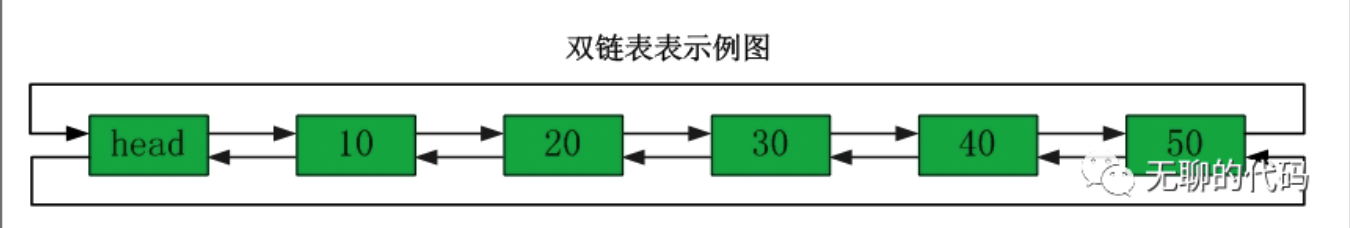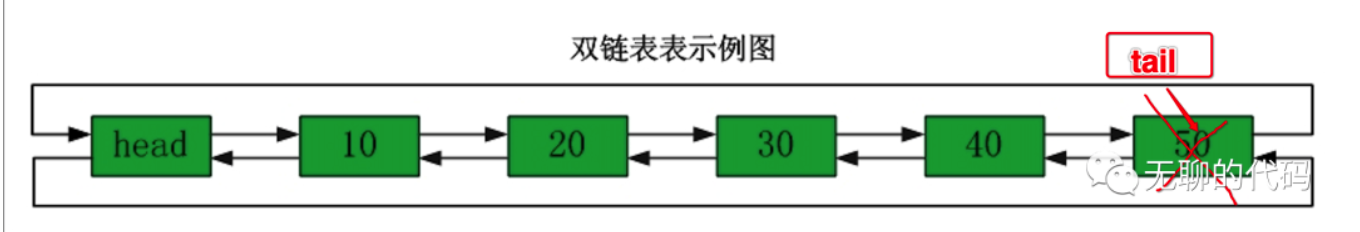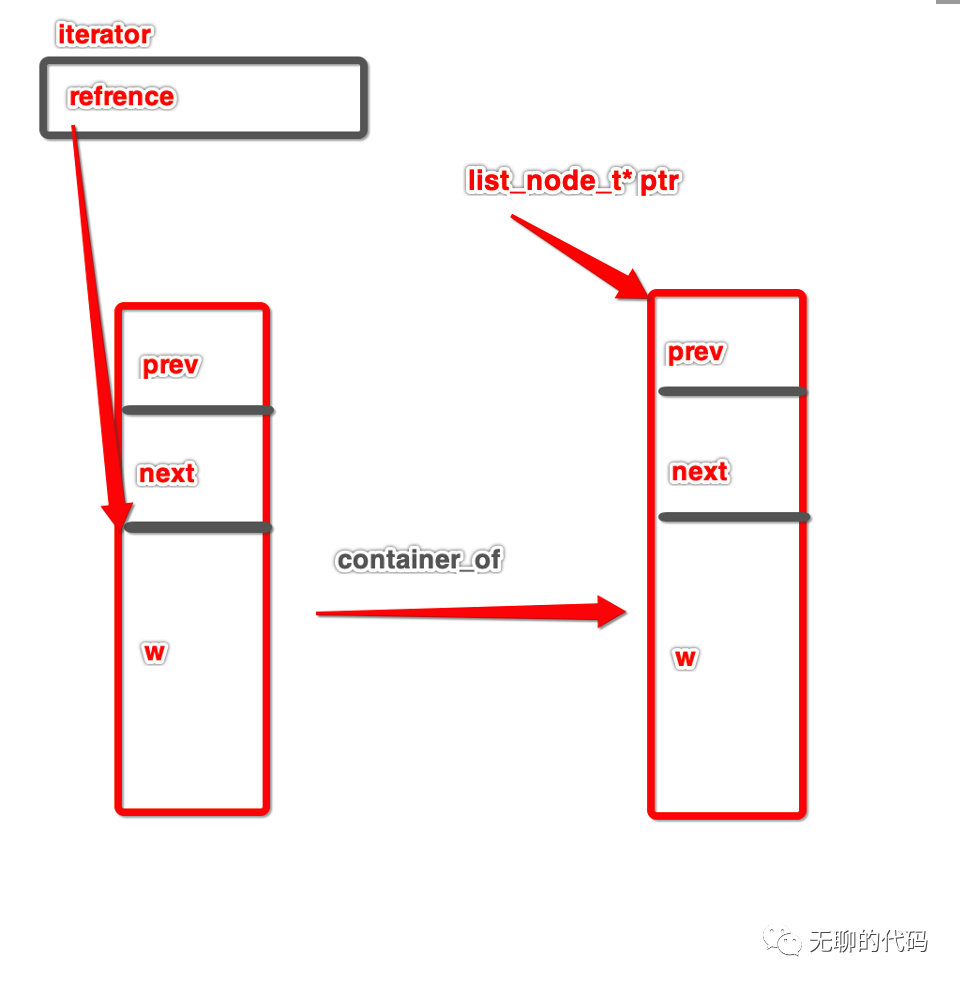# 如何实现一套容器（C 语言版）2

• 2022 年 6 月 24 日
• 本文字数：2930 字

阅读完需：约 10 分钟01 链表的设计02 代码实现

• 链表的节点定义如下：

typedef struct _list_node list_node_t;struct _list_node{    // 前一个节点的指针    list_node_t* prev;    // 后一个节点的指针    list_node_t* next;    // 数据内存，这里并不是值只有 1 个字节。    // 这个节点大小需要根据存入的数据大小来申请内存。    T w;};

• 链表的定义如下：

typedef struct _list {    // 这里可以看作继承 container 这个基类    container_t container;    list_node_t _sentinel;    int _size;    } list_t;

• 链表的 first 接口的实现：

static iterator_t __list_first (container_t* plist){    return __iterator(list_first(plist)->w, plist);}

• 链表的 last 接口实现：

static iterator_t __list_last (container_t* plist){    return __iterator(list_last(plist)->w, plist);}

• 链表的迭代器移动接口实现：

static int __list_move(iterator_t* it, int step){    list_node_t* pnode = container_of(it->reference, list_node_t, w);    for (int next = step; next; next = step > 0? next - 1: next + 1) {        if (step > 0) pnode = pnode->next;        else if (step < 0) pnode = pnode->prev;    }    it->reference = pnode->w;    return 0;}

#define offset_of(TYPE, MEMBER) \           ((char*)&(((TYPE *)0)->MEMBER))           #define container_of(ptr, TYPE, MEMBER) \({ \    TYPE* type_ptr =  (((char*)ptr) - offset_of(TYPE, MEMBER)); \    type_ptr; \})

container_of 计算过程如下:• 链表的插入实现：

static void* __list_insert(container_t* container, iterator_t pos，...){    T data[T_size(container->type_class)];        //从不定参数中读取要插入的数据。    va_list valist;    va_start(valist, pos);    // 此处调用数据对象对应的 不定参数转换函数。    // 把不定参数的值转换到 data 上。    T_vargs(container)(valist, data);    va_end(valist);        list_node_t* insert = container_of(pos.reference, list_node_t, w);        // 节点的大小根据容器中数据对象的大小申请内存    list_node_t* pnew \        = allocate(container->mem_pool, sizeof(list_node_t) \        + T_size(container->type_clazz));    // 赋值   T_setup(container->type_clazz)(pnew->w, data, 0);    // 插入    pnew->prev = insert->prev;    pnew->next = insert;    insert->prev->next = pnew;    insert->prev = pnew;    list_t *plist = container;    plist->_size++;    return 0;}

// int 型的容器container_insert(list, 1);// float 型的容器container_insert(list, 5.4);

• 链表删除

static int __list_remove(container_t* container, iterator_t pos, void* rdata){    // 删除    // 同样需要 container_of 的技术    list_node_t* remove = container_of(pos.reference, list_node_t, w);            remove->prev->next = remove->next;    remove->next->prev = remove->prev;    // 将要删除的值返回出去。    //if (rdata) container->type_def.ty_adapter.bit_cpy(rdata, remove->w);    if (rdata) type_value_cpy(rdata, remove->w, T_size(container->type_clazz));    // 回收    deallocate(container->mem_pool, remove);    ((list_t*)container)->_size--;    return 0;}

• 链表初始化

container_t* list_create(int ty) {    T_clazz __t = T_get(ty);        list_t* list = (list_t*) malloc( sizeof(list_t));    initialize_container(        list,         __list_first,         __list_last,         __list_move,        __list_insert,         __list_remove,         __t,    );    list_first(list) = list_head(list);    list_last(list) = list_tail(list);    list->_size = 0;    return list;}

03 测试

int main (){  // 测试 int 类型的容器。  list_t* list = list_create(int_t);  container_insert(list, 1);  container_insert(list, 2);  container_insert(list, 3);  container_insert(list, 4);  container_insert(list, 5);    // 输出容器内的整形 1，2，3，4，5  for (iter it = container_first(list);      !iter_eqaul(it, container_tail(list);      iter_next(it)) {            printf("%d ", iter_drefer(it);  }    // 测试 float 的类型的容器。  list_t list2 = list_create(fl_t);  container_insert(list2, 1.1);  container_insert(list2, 2.2);  container_insert(list2, 3.3);  container_insert(list2, 4.4);  container_insert(list2, 5.5);    // 输出容器内的整形 1.1，2.2，3.3，4.4，5.5  for (iter it = container_first(list);      !iter_eqaul(it, container_tail(list);      iter_next(it)) {            printf("%d ", iter_drefer(it);  }}## 评论